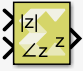# Polar to Complex - 2022.1 English

## Vitis Model Composer User Guide (UG1483)

Document ID
UG1483
Release Date
2022-05-26
Version
2022.1 English

Element-wise conversion of real magnitude and angle representation signals into a complex signal

## Library

Math Functions / Math Operations## Description

The Polar to Complex block accepts a real signal of floating point data type such as double, single, or half. The first and second inputs represent magnitude and angle respectively. The angle is in radians. The outputs are complex values of the same data type as the block input for a given real magnitude and angle. The input can be scalar, vector or matrix of real signals, in which case the output signals are also scalar, vector, or matrix. The elements of a certain magnitude input map to the magnitudes of the corresponding complex output elements. Similarly, the elements of a certain angle input map to the angles of the corresponding complex output elements.

## Data Type Support

Data types accepted at the inputs of the block are:

• Dimension : The inputs can be scalar, array, or combination of scalar and an array. If both the inputs are arrays, they must have the same dimensions.
• Data Types:  Supports signal of floating point data type such as double, single, and half. Both inputs must have the same data type.
• Complex Number Support: No

Outputs for the different input types are:

Table 1. Input/Output
Inputs Output
Both are scalar Scalar
Both are vector Vector of same dimension
Both are matrix Matrix of same dimension
One is scalar and the other is vector or matrix Dimension is that of the vector or matrix

## Parameters

The Polar to Complex block has no parameters to set.